# Latent Variable Tutorial¶

Are you building a Bayesian Network (BN) and facing one of the two situations below?

1. You wish to include a very important variable in your model but the amount of high quality data you have about it is extremely low?

2. Or you wish to include a variable for which you have no data at all, but have significant quantitative knowledge about the dependencies of this variable and the rest of the model?

In this tutorial, we show step by step how to add such a variable to your model. Here we shall focus on the interpretability aspect, i.e., we wish to learn condition probability distribution (CPD) parameters that actually represent the latent variable (LV) that we wish to include. This is an overall problem in Latent Variable Model (LVM) and we establish mechanisms to tackle this problem.

Note: To maintain code simplicity, we encapsulate some of the utility functions in tutorial_utils.py and make use of these functions in this tutorial.

Several reasons may motivate us to try to include an LV, for example:

• Obtaining true causality - We know from expert knowledge that adding an LV may create a confounding effect on the existing variables. For example, consider a simple structure below: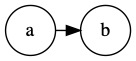Adding a confounding LV $$Z$$ might better capture the causal relationship between variables $$a$$ and $$b$$: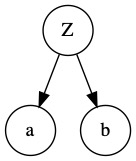• Improving performance - Including an LV changes the BN structure and can improve performance. Depending on how the LV is included, this may reduce the number of parameters and avoid overfitting, or otherwise increase the number of parameters and make the model more complex. Consider a structure below, where all parent nodes are directly connected to all child nodes: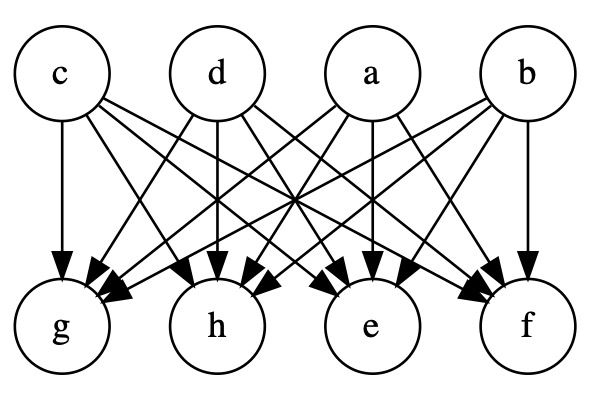Introducing an LV $$Z$$ in between the parents and children helps reduce the number of parameters and mitigate overfitting: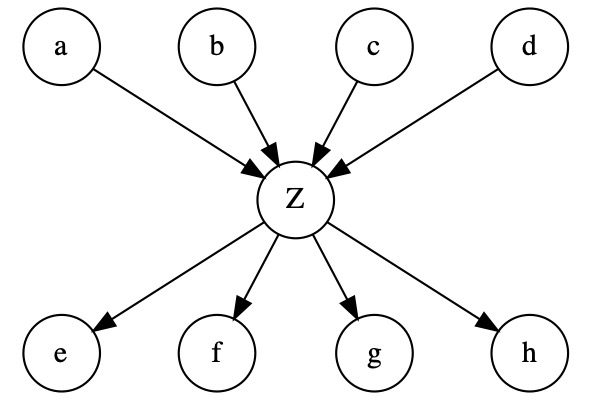### Overview of steps¶

In this tutorial, we will manually include an LV $$Z$$ in the BN structure and then use the Expectation-Maximization (EM) algorithm to learn the parameters related to this variable. This involves several steps:

Step

Description

Remark

1

Build a network with no LV and train it on complete data

We fit the CPD parameters before introducing the latent variable

2

Identify an LV and how it interacts with the model

We should specify which dependencies should be introduced and which ones should be removed

3

Add the LV to the model

Use the method BayesianNetwork.add_node to add edges to/from this LV and to remove variables that are now explained by the LV. This ensures the graph remains causal and the LV has the desired meaning. Guidelines on which sets of edges to add/remove are provided in the Appendix

4

Establish constraints to the CPDs related to the LV

Use the methods EMSingleLatentVariable.get_default_priors and EMSingleLatentVariable.get_default_box to provide constraints based on expert knowledge and little amount of data available, respectively. This would in turn help the learned parameters converge to those that the user desires

5

Fit the CPDs related to the LV using the EM algorithm

Use the method BayesianNetwork.fit_latent_cpds to learn/update the parameters of the LV and its children

### Expectation-Maximization¶

The Expectation-Maximization (EM) algorithm is an iterative method to find the Maximum Likelihood Estimator (MLE) or Maximum a Posteriori (MAP) in the presence of latent variables. It is a general algorithm, not restricted only to Bayesian Networks. It works by alternating between two steps:

• Expectation (E-step) - Given the current state of model parameters, we estimate the expected log likelihood. This expected value takes into account all the values the LV can assume and their probabilities. More formally, if $$Z$$ is the latent variable, $$\theta$$ is the current parameters, and $$X$$ is the realisations of the observed of variables, we compute:

$$Q(X|\theta) = \int \log P(X,Z|\theta) dZ$$

Note that $$Q$$ is a lower bound for the model log likelihood. Based on the Jensen inequality $$f(E[X]) \geq E[f(X)]$$ for concave function $$f$$ (including $$\log$$ in this case), we can get the following:

$$L(X|\theta) = \log P(X|\theta) = \log \int P(X,Z|\theta)dZ \geq Q(X|\theta)$$

• Maximisation (M-step) - Update the parameters to maximise the expectation computed in the previous step:

$$\arg \max_{\theta} Q(X|\theta)$$

For interested readers, please refer to the original EM work at 

#### Guarantees and limitations¶

EM comes with guarantees and limitations. The main guarantees are:

• Successive steps always improve the likelihood

• It converges to a local maxima in the likelihood function

We also address several limitations below:

• Interpretability

• It is difficult to ensure that the meaning of the LV is as desired. For example, EM might learn the parameters for a different variable than the one we desire.

• To address this, we can either provide some data about the LV (in many cases a few samples may suffice) or impose constraints on the parameters. The constraints currently allowed in our implementation will be covered below.

• Computational complexity

• Because we need to consider all values of the LV in the E-step, each iteration is slower than MLE due to extra (linear) complexity associated with the cardinality of the LV.

• Also, as many iterations may be needed for convergence, the algorithm can be slow for large datasets. The current implementation may scale up to few tens of thousands of data points, but there may be room for further optimisation.

#### Our implementation¶

Our EM implementation in CausalNex is captured in the EMSingleLatentVariable class, which is invoked from the fit_latent_cpds method under the BayesianNetwork class.

EMSingleLatentVariable runs the EM algorithm in order to learn the CPDs of a specified LV along with its children/successors. This is based on the property outlined here.

#### Constraints¶

It is important to note that we can and should provide constraints to the learned parameters in three different ways:

• Provide some quantity of data (in many cases 50-100 samples would be enough)

• Use argument box_constraints to constrain each CPD parameter to be inside a given interval (say, $$P(A=1|B=0,C=1) \in [0.1, 0.5]$$)

• Use argument priors to provide specific Dirichlet priors

These constraints are very important if we want to interpret the meaning of our latent variable. Without them, EM could converge to a local maximum representing a different unobserved variable.

### Case study: Student performance data¶

In this case study, we demonstrate an application of EM to the student performance dataset from UCI Machine Learning Repository. In particular, you will need download the student.zip file and extract student-por.csv from the zip file into the same directory as this notebook.

:

%load_ext autoreload

:

import logging
import warnings
import numpy as np
import pandas as pd
import tutorial_utils as utils

logging.basicConfig(level=logging.ERROR)
warnings.filterwarnings("ignore")
pd.options.display.max_columns = 100


We assume that we have NO DATA about the students’ grade on the first exam of the semester, i.e., G1. We try to recover this variable with the assumption that students tend to perform similarly in two successive exams.

In the original dataset, this variable is provided. We keep this in g1_column to check the accuracy of our solution later.

:

df = pd.read_csv('student-por.csv', delimiter=";")
g1_column = df["G1"]
df["G1"] = np.nan
print("dataset rows and columns: ", df.shape)

dataset rows and columns:  (649, 33)

:

school sex age address famsize Pstatus Medu Fedu Mjob Fjob reason guardian traveltime studytime failures schoolsup famsup paid activities nursery higher internet romantic famrel freetime goout Dalc Walc health absences G1 G2 G3
0 GP F 18 U GT3 A 4 4 at_home teacher course mother 2 2 0 yes no no no yes yes no no 4 3 4 1 1 3 4 NaN 11 11
1 GP F 17 U GT3 T 1 1 at_home other course father 1 2 0 no yes no no no yes yes no 5 3 3 1 1 3 2 NaN 11 11
2 GP F 15 U LE3 T 1 1 at_home other other mother 1 2 0 yes no no no yes yes yes no 4 3 2 2 3 3 6 NaN 13 12
3 GP F 15 U GT3 T 4 2 health services home mother 1 3 0 no yes no yes yes yes yes yes 3 2 2 1 1 5 0 NaN 14 14
4 GP F 16 U GT3 T 3 3 other other home father 1 2 0 no yes no no yes yes no no 4 3 2 1 2 5 0 NaN 13 13

The structure we started with does not contain the variable G1. In the section below, we highlight the edges that we will remove when adding G1 to the model.

:

from causalnex.plots import plot_structure
from IPython.display import Image

"./supporting_files/student.dot",
"G1",
)
viz = utils.plot_pretty_structure(sm, edges_to_highlight=g1_edges_to_remove)
Image(viz.draw(format='png'))

:#### Building a network with no LV and train it on complete data¶

Below we learn the network CPDs for the structure model without G1.

:

from causalnex.network import BayesianNetwork

bn = BayesianNetwork(sm)

# Dropping VehicleYear because the fit_node_states does not accept missing values
bn.fit_node_states_and_cpds(df.drop(['G1'], axis=1))

:

<causalnex.network.network.BayesianNetwork at 0x154d9a070>


#### Identifying an LV and how it interacts with the model¶

We need to carefully identify what dependencies this variable would have in the system. Here we identify G1 as LV and insert it in between G2 and its parents.

To this end, we specify new edges from/to G1, as well as edges to be removed between G2 and its parents.

:

print("We are going to add:")
print("\nWe are going to remove:")
print(g1_edges_to_remove)

We are going to add:
[('school', 'G1'), ('G1', 'G2'), ('address', 'G1'), ('studytime', 'G1'), ('failures', 'G1'), ('higher', 'G1')]

We are going to remove:
[('school', 'G2'), ('address', 'G2'), ('studytime', 'G2'), ('failures', 'G2'), ('higher', 'G2')]


#### Adding the LV to the network¶

We can now add the LV G1 into our network as follows.

:

bn.add_node(
node="G1",
edges_to_remove=g1_edges_to_remove,
)

:

<causalnex.network.network.BayesianNetwork at 0x154d9a070>

:

viz = utils.plot_pretty_structure(bn.structure, edges_to_highlight=g1_edges_to_add)
Image(viz.draw(format='png'))

:#### Fitting the parameters of the LV and its children¶

We do not need to refit (re-learn) all the CPD parameters of all nodes in the BN. Based on this property, only a small number of parameters need to be learned: the CPD of LV and that of its children.

:

# This takes several minutes...
bn.fit_latent_cpds(
lv_name="G1",
lv_states=[0, 4, 5, 6, 7, 8, 9, 10, 11, 12, 13, 14, 15, 16, 17, 18, 19],
data=df,
box_constraints=boundaries,
priors=priors,
n_runs=30,
)

:

<causalnex.network.network.BayesianNetwork at 0x154d9a070>

##### Querying the updated model¶

We can now use the updated BN. Below is a simple query from CausalNex’s inference engine.

:

from causalnex.inference import InferenceEngine

ie = InferenceEngine(bn)
ie.do_intervention('studytime', 3)
query = ie.query()

print("P(G1=10|do(studytime=3)) = ", query["G1"])
print("P(G2=10|do(studytime=3)) = ", query["G2"])

P(G1=10|do(studytime=3)) =  0.005380615673278852
P(G2=10|do(studytime=3)) =  0.09461209113528016

##### Checking the accuracy of the parameters learned¶

Since the missing data was introduced rather than being there a priori, we can simply complete the missing data, fit the parameters with MLE, and compare the CPDs learned by the two methods.

If we compute the absolute error in every single parameter, we can see that the average absolute error is of 0.05. This means that:

The parameters learned in an almost unsupervised fashion are very close to the ones learned with the full dataset.

:

bn_check = BayesianNetwork(bn.structure)
df_complete = df.copy(deep=True)
df_complete["G1"] = g1_column

bn_check.fit_node_states_and_cpds(df_complete)
vals = np.concatenate(
tuple(
np.abs(bn.cpds[el] - bn_check.cpds[el]).values.flatten()
for el in ['G1','G2']
)
)
print(vals.mean())

0.049378397638738955

:

import matplotlib.pyplot as plt

fig, ax = plt.subplots(1, 1, figsize=(10, 3))
ax.hist(vals)
ax.set_title('Distribution of the absolute errors on the parameters')
fig.tight_layout()
fig.show()## Identifying candidate locations for latent variable¶

The earlier section assumes that we already know the location in the graph of the LV we wish to include. Sometimes, however, we may not be very certain about its location in our BN. Whilst data cannot provide a definitive answer, in this post we explore strategies to identify “candidate locations” for LVs that can help augment domain knowledge.

### Overview of steps¶

Evaluating our approach based on existing data observations (which have no strict ‘ground truth’ per se) is tricky. In this tutorial, we present the following evaluation procedure:

1. Learn the conditional probability distribution (CPD) of every node in the BN without adding any LV

2. Estimate the average AUC of each node (treating that node as the target variable) using k-fold cross validation and use that as a baseline

3. Add the LV using a location identification strategy, and learn the CPD of the LV and its parents using the EM algorithm presented earlier in this tutorial

4. Re-calculate the average AUC of each node using k-fold cross-validation

5. Compare the newly calculated AUC with the baseline in step 2

### Location identification strategy¶

In this tutorial, we present a heuristic strategy to identify candidate LV locations by adding an LV between a pair of nodes with highly correlation score. Intuitively, nodes (variable) with high correlation are likely driven by a latent, confounding variable.

Specifically, we first find a pair of connected nodes that has the highest Spearman correlation. We can then judge whether this pair makes a causal sense, perhaps through consultation with domain experts. Once this is verified, we remove the edge between these nodes and add an LV between them accordingly.

As an illustration, consider two nodes $$a$$ and $$b$$ below, which have high Spearman correlation score and are selected through discussion with domain experts:Based on this strategy, we introduce a confounding LV $$Z$$ as follows:Note: This correlation-based strategy is only one of the many possible heuristics and, certainly, there is room of improvement using a more advanced strategy. We shall nevertheless leave this for future research. If you are interested to pursue this further, please submit your contribution as per the contribution guideline.

### Case study: Student performance data¶

We will again illustrate this strategy using the student performance dataset from UCI Machine Learning Repository.

#### Model initialisation¶

Firstly, we load the dataset and construct the baseline BN using the NOTEARS algorithm.

:

from sklearn.preprocessing import LabelEncoder
from time import time

from causalnex.discretiser import Discretiser
from causalnex.network import BayesianNetwork
from causalnex.structure.notears import from_pandas

df.drop(columns=['school','sex','age','Mjob', 'Fjob','reason','guardian'], inplace=True)

struct_data = df.copy()
non_numeric_columns = list(struct_data.select_dtypes(exclude=[np.number]).columns)
print(f'Non-numeric columns: {non_numeric_columns}')

Non-numeric columns: ['address', 'famsize', 'Pstatus', 'schoolsup', 'famsup', 'paid', 'activities', 'nursery', 'higher', 'internet', 'romantic']

:

t0 = time()
le = LabelEncoder()

for col in non_numeric_columns:
struct_data[col] = le.fit_transform(struct_data[col])

sm = from_pandas(struct_data, w_threshold=0.8)
sm = sm.get_largest_subgraph()
bn = BayesianNetwork(sm)
print(f'Running NOTEARS algorithm takes {time() - t0} seconds')

Running NOTEARS algorithm takes 55.29370975494385 seconds

:

viz = utils.plot_pretty_structure(bn.structure, edges_to_highlight=[])
Image(viz.draw(format='png'))

:Next, we discretise the data so that it is compatible with CausalNex.

:

discretised_data = struct_data.copy()
data_vals = {col: discretised_data[col].unique() for col in discretised_data.columns}

# Discretise categorical variables
failures_map = {
v: 'no-failure' if v ==  else 'have-failure' for v in data_vals['failures']
}
studytime_map = {
v: 'short-studytime' if v in [1,2] else 'long-studytime' for v in data_vals['studytime']
}
discretised_data["failures"] = discretised_data["failures"].map(failures_map)
discretised_data["studytime"] = discretised_data["studytime"].map(studytime_map)

# Discretise numeric variables
discretised_data["absences"] = Discretiser(
method="fixed", numeric_split_points=[1, 10]
).transform(discretised_data["absences"].values)
discretised_data["G1"] = Discretiser(
method="fixed", numeric_split_points=
).transform(discretised_data["G1"].values)
discretised_data["G2"] = Discretiser(
method="fixed", numeric_split_points=
).transform(discretised_data["G2"].values)
discretised_data["G3"] = Discretiser(
method="fixed", numeric_split_points=
).transform(discretised_data["G3"].values)

absences_map = {0: "No-absence", 1: "Low-absence", 2: "High-absence"}
G1_map = {0: "Fail", 1: "Pass"}
G2_map = {0: "Fail", 1: "Pass"}
G3_map = {0: "Fail", 1: "Pass"}

discretised_data["absences"] = discretised_data["absences"].map(absences_map)
discretised_data["G1"] = discretised_data["G1"].map(G1_map)
discretised_data["G2"] = discretised_data["G2"].map(G2_map)
discretised_data["G3"] = discretised_data["G3"].map(G3_map)


#### Establishing baseline performance¶

We are now ready to fit the node states based on the discretised data.

:

bn.fit_node_states(discretised_data)

:

<causalnex.network.network.BayesianNetwork at 0x1552d5430>


Subsequently, we can use CausalNex’s inference engine to query the marginals given the value of other variables. That is, we calculate $$P(node|G_{node})$$ where $$G_{node}$$ includes every variable in the graph other than the target node and LV. The utility function tutorial_utils.get_avg_auc_all_info encapsulates this inference mechanism, and computes the average AUC using k-fold cross validation.

:

baseline_auc = utils.get_avg_auc_all_info(discretised_data, bn)
print(f"Baseline AUC: {baseline_auc}")

Processing fold 0 using 15 cores takes 13.75121283531189 seconds
Processing fold 1 using 15 cores takes 14.090878009796143 seconds
Processing fold 2 using 15 cores takes 13.760735988616943 seconds
Processing fold 3 using 15 cores takes 14.692227125167847 seconds
Processing fold 4 using 15 cores takes 14.706875085830688 seconds
Baseline AUC: 0.8461702489162135


#### Identifying highly correlated nodes¶

The next step is to extract pairs of adjacent nodes and choose the pair with the highest score. It is important to note that there should be an edge from one node to the other.

:

correl_df = utils.adjacency_correlations(struct_data, bn, 'spearman')
utils.display_colored_df(correl_df)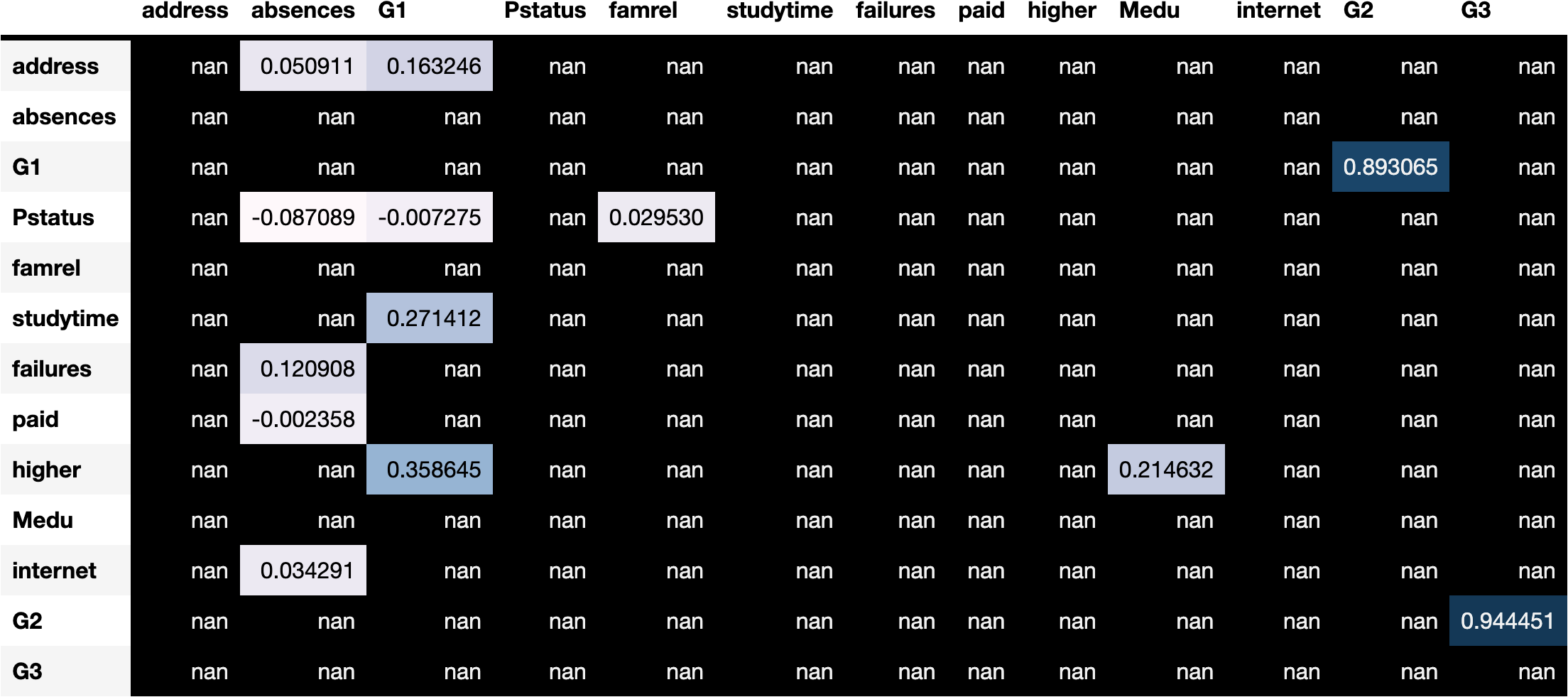As we can see here, G2 and G3 has a Spearman score of 0.94 and G2 is the parent of G3. Therefore, based on our strategy, we will place an LV between those two nodes.

:

import copy

edges_to_add = [('LV', 'G2'), ('LV', 'G3')]
edges_to_remove = [('G2', 'G3')]

bn_with_lv = copy.deepcopy(bn)
"LV",
edges_to_remove=edges_to_remove,
)

:

<causalnex.network.network.BayesianNetwork at 0x157c4cd00>

:

viz = utils.plot_pretty_structure(bn_with_lv.structure, edges_to_highlight=edges_to_add)
Image(viz.draw(format='png'))

:#### Comparison with baseline performance¶

Finally, we can compare the performance of the model with the addition of the LV and that of the base/original model.

:

discretised_data['LV'] = None
lv_states = [0, 1, 2, 3, 4]

proposed_auc = utils.get_avg_auc_lvs(discretised_data, bn_with_lv, lv_states)
print(f"AUC from adding LV between 'G2' and 'G3': {proposed_auc}")

Processing fold 0 using 15 cores takes 14.323214769363403 seconds
Processing fold 1 using 15 cores takes 15.189436197280884 seconds
Processing fold 2 using 15 cores takes 15.276448965072632 seconds
Processing fold 3 using 15 cores takes 15.079718828201294 seconds
Processing fold 4 using 15 cores takes 15.241908073425293 seconds
AUC from adding LV between 'G2' and 'G3': 0.8566012338312075


With an AUC score of 0.857, our new model has outperformed the baseline model, which indicates that the LV is a worthy addition to the graph.

## Appendix¶

### How to choose where to add an LV?¶

One should always add LV edges based on the known true causality relationship.

However, certain configurations allow EM to more likely converge to the correct CPD parameters of the LV. Other configurations make it more difficult for EM to learn the correct parameters and may create misleading results.

Understanding these configurations is therefore crucial to adding latent variables to a BN.

### An interesting property of EM for Bayesian networks¶

Applying EM for Bayesian Networks in our current setting (i.e. no missing data except for the LV itself) has the following property:

The only parameters that need to be learned through EM are the CPDs <./05_latent_variable_tutorial.html#Definitions>__ of the LV itself and its children. The other parameters CPDs can be learned directly using MLE.

This property comes directly from the conditional independence on which the BN is based.

From this property, we can limit ourselves to understanding the behaviour of EM given the LV’s Markov blanket. We detail the different possible situations we can face in the next section.

### What structures allow EM to correctly recover parameters?¶

In order for EM to learn meaningful parameters, there has to be some correlation between variables that is not explained by the edges among observed variables.

A few cases in which EM works well:

1. In the naive Bayes structure below, EM can work well because a possible correlation between the observed variables can only be explained by a causality of the LV ($$Z$$)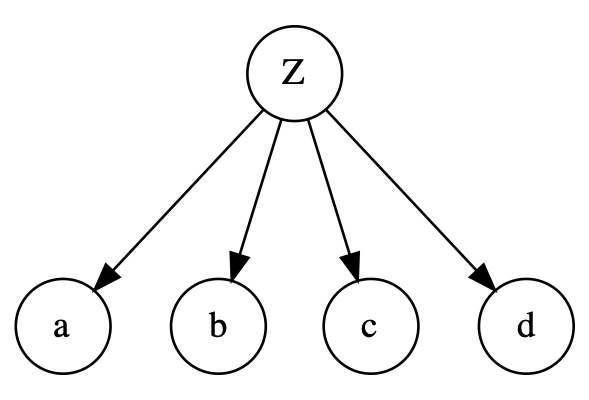1. In the following structure, $$Z$$ explains the correlations among the nodes on the bottom, and also the correlation between nodes on top and the ones on the bottom. It is likely that we are able to recover the correct parameters in this situation.Other cases where EM is not likely to provide meaningful parameters:

1. When the LV has no children, it would be quite difficult to infer its true parameters. EM will still converge but not necessarily to a meaningful result.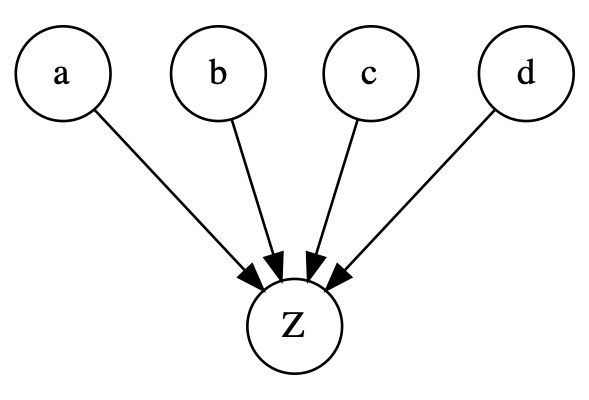1. Below we have a structure whereby the children are fully connected. In this case, even if, in reality, the unobserved variable affects all children, all the correlations could be derived from the existing edges. The edge $$e \rightarrow f$$ explains the correlation between $$a$$ and $$b$$ for example. Therefore, EM would likely not retrieve the correct parameters for $$Z$$.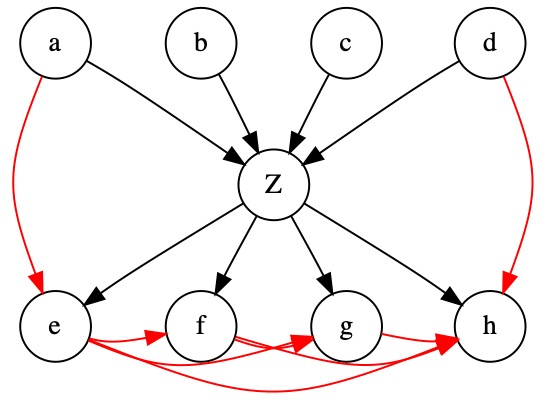### Definitions¶

 A CPD of a node $$X$$ is a table specifying the probability $$P(X|Parents(X))$$. In a discrete Bayesian Network, these are provided as tables. The parameters of a BN are the set of the entries in every node.

 A Markov Blanket of a node is defined as the set of its parents, its children and its parent’s children